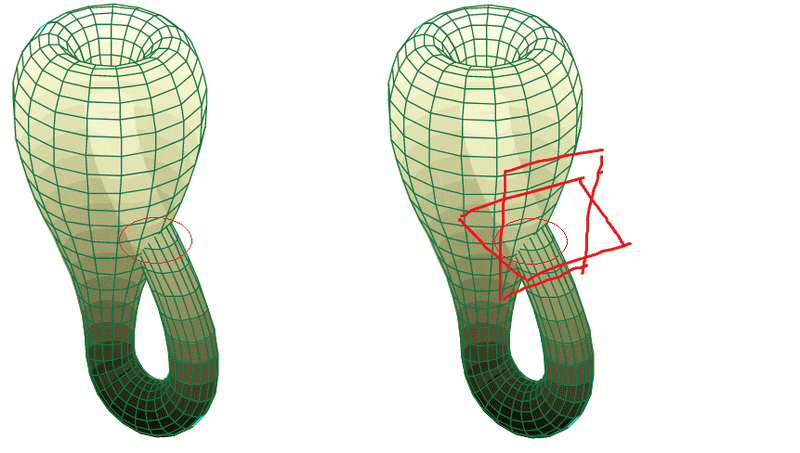# Klein Bottle immersion test

I know that there exists non-geometrical proofs that the usual mapping of the Klein Bottle in ##\mathbb{R}^3## is an immersion. But I would like to see an actual geometrical 'test'. I was thinking if saying that on the self intersection, which I circled in red below, the map being injective can be justified by saying that it's because there are two distinct tangent spaces, which is drawn in the right picture?#### Attachments

lavinia
Gold Member
I know that there exists non-geometrical proofs that the usual mapping of the Klein Bottle in ##\mathbb{R}^3## is an immersion. But I would like to see an actual geometrical 'test'. I was thinking if saying that on the self intersection, which I circled in red below, the map being injective can be justified by saying that it's because there are two distinct tangent spaces, which is drawn in the right picture?
View attachment 227447
The tangent planes do not need to be distinct in an immersion. An immersion must be smooth so that there must be a well defined tangent plane in the image of any point.

The picture of the Klein bottle above is an immersion because along every part of the tube there is a tangent plane. At the intersection of the tube with itself there are two planes each coming from another part of the Klein bottle. But in theory these planes could be the same.

If the map had a crease or a cusp or crushed a line to a point then it would not be an immersion

Last edited:
•Klystron and FactChecker
in theory these planes could be the same
do you mean we can rotate one of those planes I have drawn to the other's direction so that they become same? But wouldn't that make your statement
At the intersection of the tube with itself there are two planes each coming from another part of the Klein bottle
meaningless?

lavinia
Gold Member
do you mean we can rotate one of those planes I have drawn to the other's direction so that they become same? But wouldn't that make your statement

meaningless?
The two parts of the tube could kiss for a while before parting directions. At the points where they kiss the tangent planes would be the same. Since it must at some point pass through itself I imagine that the tangent planes must be distinct at some point along the intersection circle - but not sure.

The map ##x→e^{ix}## is an immersion of the real line into the complex plane onto the unit circle. Each point on the circle is hit infinitely many times ,each time with the same tangent line.

Last edited:
martinbn
Kent, may be you are being confused about immersion and embedding.

Kent, may be you are being confused about immersion and embedding.
No, I'm really considering the immersion.
Since it must at some point pass through itself I imagine that the tangent planes must be distinct at some point along the intersection circle - but not sure.
Yes, but I think this always happens, so cannot be used as a way to see if it's an immersion. The tangent planes being different after the intersection is a consequence of the end of the self intersection, i.e. the map is injective again, correct?
The map ##x→e^{ix}## is an immersion of the real line into the complex plane onto the unit circle. Each point on the circle is hit infinitely many times ,each time with the same tangent line.
Unfortunately, I do not think this is a good example, because as far as I know any one dimensional manifold can be immersed in a higher dimensional manifold. Also, any point of ##\mathbb{R}## has the same trivial tangent space, which is ##\mathbb{R}## itself. So the only way is the circle being hit infinitely many times by the same tangent line.On the other hand, the Klein bottle is a 2-dimensional manifold, so I guess we would need a 2-dimensional known case to use as an example.

lavinia
Gold Member
Yes, but I think this always happens, so cannot be used as a way to see if it's an immersion. The tangent planes being different after the intersection is a consequence of the end of the self intersection, i.e. the map is injective again, correct?

It is the existence of tangent planes that makes it an immersion.

Unfortunately, I do not think this is a good example, because as far as I know any one dimensional manifold can be immersed in a higher dimensional manifold. Also, any point of ##\mathbb{R}## has the same trivial tangent space, which is ##\mathbb{R}## itself. So the only way is the circle being hit infinitely many times by the same tangent line.On the other hand, the Klein bottle is a 2-dimensional manifold, so I guess we would need a 2-dimensional known case to use as an example.

It is a good example. If you insist on a higher dimensional example consider the immersion of ##R^2## into ##R^4## onto the flat torus ##(x,y)→(e^{ix},e^{iy})##

BTW:
Any manifold can be immersed in some higher dimensional manifold.

Any covering transformation is an immersion. So there is an immersion of ##R^2## onto the Klein bottle. Each point on the Klein bottle is hit infinitely many times and always with the same tangent plane.

Last edited:
•kent davidge